# Tag: profinite

Sunday january 2nd around 18hr NeB-stats went crazy.

Referrals clarified that the post ‘What is the knot associated to a prime?’ was picked up at Reddit/math and remained nr.1 for about a day.

Now, the dust has settled, so let’s learn from the experience.A Reddit-mention is to a blog what doping is to a sporter.

You get an immediate boost in the most competitive of all blog-stats, the number of unique vistors (blue graph), but is doesn’t result in a long-term effect, and, it may even be harmful to more essential blog-stats, such as the average time visitors spend on your site (yellow graph).

For NeB the unique vistors/day fluctuate normally around 300, but peaked to 1295 and 1733 on the ‘Reddit-days’. In contrast, the avg. time on site is normally around 3 minutes, but dropped the same days to 44 and 30 seconds!

Whereas some of the Reddits spend enough time to read the post and comment on it, the vast majority zap from one link to the next. Having monitored the Reddit/math page for two weeks, I’m convinced that post only made it because it was visually pretty good. The average Reddit/math-er is a viewer more than a reader…

So, should I go for shorter, snappier, more visual posts?

Let’s compare Reddits to those coming from the three sites giving NeB most referrals : Google search, MathOverflow and Wikipedia.This is the traffic coming from Reddit/math, as always the blue graph are the unique visitors, the yellow graph their average time on site, blue-scales to the left, yellow-scales to the right.

Here’s the same graph for Google search. The unique visitors/day fluctuate around 50 and their average time on site about 2 minutes.The math-related search terms most used were this month : ‘functor of point approach’, ‘profinite integers’ and ‘bost-connes sytem’.

More rewarding to me are referrals from MathOverflow.The number of visitors depends on whether the MathO-questions made it to the front-page (for example, the 80 visits on december 15, came from the What are dessins d’enfants?-topic getting an extra comment that very day, and having two references to NeB-posts : The best rejected proposal ever and Klein’s dessins d’enfant and the buckyball), but even older MathO-topics give a few referrals a day, and these people sure take their time reading the posts (+ 5 minutes).

Other MathO-topics giving referrals this month were Most intricate and most beautiful structures in mathematics (linking to Looking for F-un), What should be learned in a first serious schemes course? (linking to Mumford’s treasure map (btw. one of the most visited NeB-posts ever)), How much of scheme theory can you visualize? (linking again to Mumford’s treasure map) and Approaches to Riemann hypothesis using methods outside number theory (linking to the Bost-Connes series).

Finally, there’s Wikipediagiving 5 to 10 referrals a day, with a pretty good time-on-site average (around 4 minutes, peaking to 12 minutes). It is rewarding to see NeB-posts referred to in as diverse Wikipedia-topics as ‘Fifteen puzzle’, ‘Field with one element’, ‘Evariste Galois’, ‘ADE classification’, ‘Monster group’, ‘Arithmetic topology’, ‘Dessin d’enfant’, ‘Groupoid’, ‘Belyi’s theorem’, ‘Modular group’, ‘Cubic surface’, ‘Esquisse d’un programme’, ‘N-puzzle’, ‘Shabat polynomial’ and ‘Mathieu group’.

What lesson should be learned from all this data? Should I go for shorter, snappier and more visual posts, or should I focus on the small group of visitors taking their time reading through a longer post, and don’t care about the appallingly high bounce rate the others cause?

Sometimes a MathOverflow question gets deleted before I can post a reply…

Yesterday (New-Year) PD1&2 were visiting, so I merely bookmarked the What is the knot associated to a prime?-topic, promising myself to reply to it this morning, only to find out that the page no longer exists.

From what I recall, the OP interpreted one of my slides of the April 1st-Alumni talk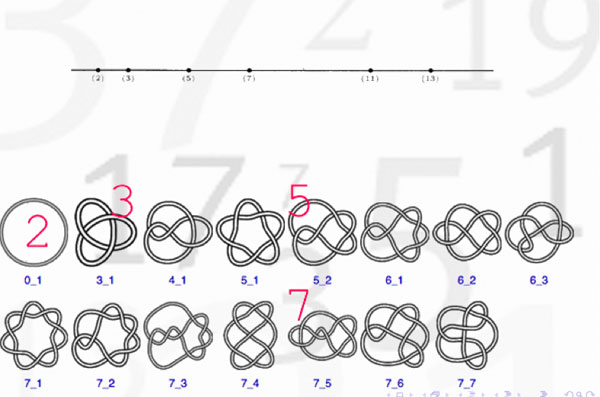as indicating that there might be a procedure to assign to a prime number a specific knot. Here’s the little I know about this :

Artin-Verdier duality in etale cohomology suggests that $Spec(\mathbb{Z})$ is a 3-dimensional manifold, as Barry Mazur pointed out in this paper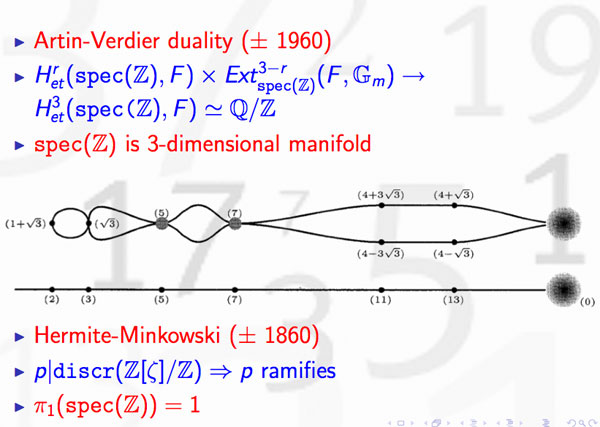The theory of discriminants shows that there are no non-trivial global etale extensions of $Spec(\mathbb{Z})$, whence its (algebraic) fundamental group should be trivial. By Poincare-Perelman this then implies that one should view $Spec(\mathbb{Z})$ as the three-sphere $S^3$. Note that there is no ambiguity in this direction. However, as there are other rings of integers in number fields having trivial fundamental group, the correspondence is not perfect.

Okay, but then primes should correspond to certain submanifolds of $S^3$ and as the algebraic fundamental group of $Spec(\mathbb{F}_p)$ is the profinite completion of $\mathbb{Z}$, the first option that comes to mind are circles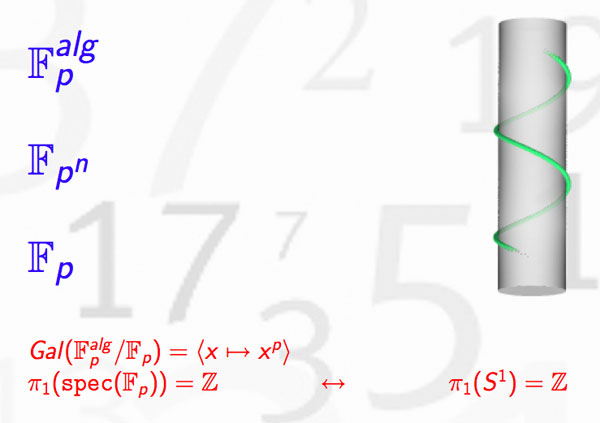Hence, primes might be viewed as circles embedded in $S^3$, that is, as knots! But which knots? Well, as far as I know, nobody has a procedure to assign a knot to a prime number, let alone one having p crossings. What is known, however, is that different primes must correspond to different knots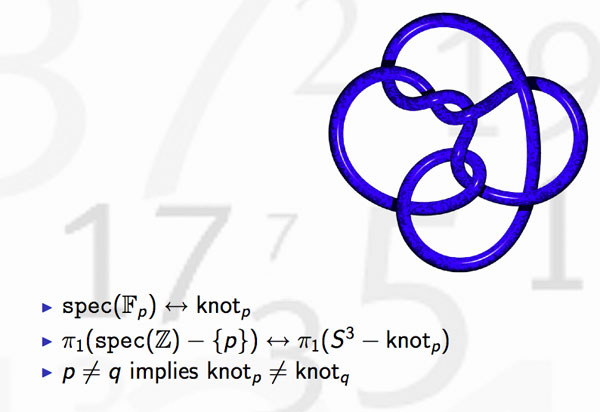because the algebraic fundamental groups of $Spec(\mathbb{Z})- { p }$ differ for distinct primes. This was the statement I wanted to illustrate in the first slide.

But, the story goes a lot further. Knots may be linked and one can detect this by calculating the link-number, which is symmetric in the two knots. In number theory, the Legendre symbol, plays a similar role thanks to quadratic reciprocity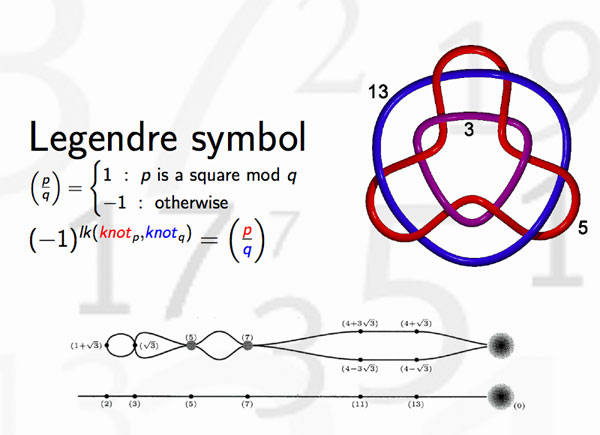and hence we can view the Legendre symbol as indicating whether the knots corresponding to different primes are linked or not. Whereas it is natural in knot theory to investigate whether collections of 3, 4 or 27 knots are intricately linked (or not), few people would consider the problem whether one collection of 27 primes differs from another set of 27 primes worthy of investigation.

There’s one noteworthy exception, the Redei symbol which we can now view as giving information about the link-behavior of the knots associated to three different primes. For example, one can hunt for prime-triples whose knots link as the Borromean rings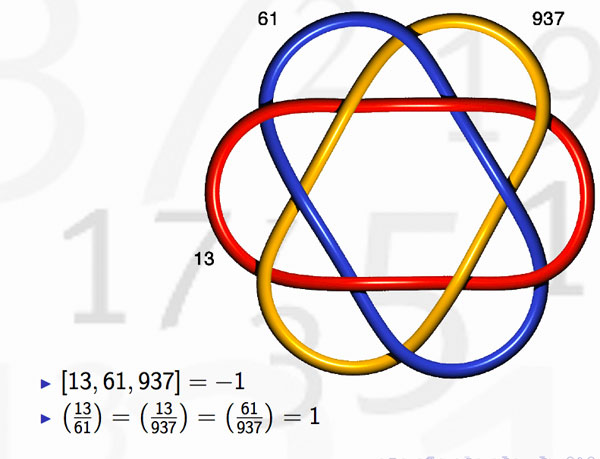(note that the knots corresponding to the three primes are not the unknot but more complicated). Here’s where the story gets interesting : in number-theory one would like to discover ‘higher reciprocity laws’ (for collections of n prime numbers) by imitating higher-link invariants in knot-theory. This should be done by trying to correspond filtrations on the fundamental group of the knot-complement to that of the algebraic fundamental group of $Spec(\mathbb{Z})-{ p }$ This project is called arithmetic topology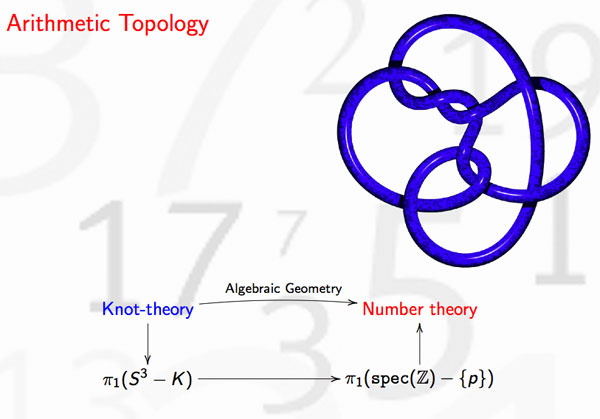Perhaps I should make a pod- or vod-cast of that 20 minute talk, one day…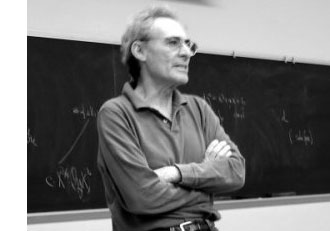In the previous posts, we have depicted the ‘arithmetic line’, that is the prime numbers, as a ‘line’ and individual primes as ‘points’.

However, sometime in the roaring 60-ties, Barry Mazur launched the crazy idea of viewing the affine spectrum of the integers, $\mathbf{spec}(\mathbb{Z})$, as a 3-dimensional manifold and prime numbers themselves as knots in this 3-manifold…

After a long silence, this idea was taken up recently by Mikhail Kapranov and Alexander Reznikov (1960-2003) in a talk at the MPI-Bonn in august 1996. Pieter Moree tells the story in his recollections about Alexander (Sacha) Reznikov in Sipping Tea with Sacha : “Sasha’s paper is closely related to his paper where the analogy of covers of three-manifolds and class field theory plays a big role (an analogy that was apparently first noticed by B. Mazur). Sasha and Mikhail Kapranov (at the time also at the institute) were both very interested in this analogy. Eventually, in August 1996, Kapranov and Reznikov both lectured on this (and I explained in about 10 minutes my contribution to Reznikov’s proof). I was pleased to learn some time ago that this lecture series even made it into the literature, see Morishita’s ‘On certain analogies between knots and primes’ J. reine angew. Math 550 (2002) 141-167.”

Here’s a part of what is now called the Kapranov-Reznikov-Mazur dictionary :What is the rationale behind this dictionary? Well, it all has to do with trying to make sense of the (algebraic) fundamental group $\pi_1^{alg}(X)$ of a general scheme $X$. Recall that for a manifold $M$ there are two different ways to define its fundamental group $\pi_1(M)$ : either as the closed loops in a given basepoint upto homotopy or as the automorphism group of the universal cover $\tilde{M}$ of $M$.

For an arbitrary scheme the first definition doesn’t make sense but we can use the second one as we have a good notion of a (finite) cover : an etale morphism $Y \rightarrow X$ of the scheme $X$. As they form an inverse system, we can take their finite automorphism groups $Aut_X(Y)$ and take their projective limit along the system and call this the algebraic fundamental group $\pi^{alg}_1(X)$.

Hendrik Lenstra has written beautiful course notes on ‘Galois theory for schemes’ on all of this starting from scratch. Besides, there are also two video-lectures available on this at the MSRI-website : Etale fundamental groups 1 by H.W. Lenstra and Etale fundamental groups 2 by F. Pop.

But, what is the connection with the ‘usual’ fundamental group in case both of them can be defined? Well, by construction the algebraic fundamental group is always a profinite group and in the case of manifolds it coincides with the profinite completion of the standard fundamental group, that is,
$\pi^{alg}_1(M) \simeq \widehat{\pi_1(M)}$ (recall that the cofinite completion is the projective limit of all finite group quotients).

Right, so all we have to do to find a topological equivalent of an algebraic scheme is to compute its algebraic fundamental group and find an existing topological space of which the profinite completion of its standard fundamental group coincides with our algebraic fundamental group. An example : a prime number $p$ (as a ‘point’ in $\mathbf{spec}(\mathbb{Z})$) is the closed subscheme $\mathbf{spec}(\mathbb{F}_p)$ corresponding to the finite field $\mathbb{F}_p = \mathbb{Z}/p\mathbb{Z}$. For any affine scheme of a field $K$, the algebraic fundamental group coincides with the absolute Galois group $Gal(\overline{K}/K)$. In the case of $\mathbb{F}_p$ we all know that this abslute Galois group is isomorphic with the profinite integers $\hat{\mathbb{Z}}$. Now, what is the first topological space coming to mind having the integers as its fundamental group? Right, the circle $S^1$. Hence, in arithmetic topology we view prime numbers as topological circles, that is, as knots in some bigger space.

But then, what is this bigger space? That is, what is the topological equivalent of $\mathbf{spec}(\mathbb{Z})$? For this we have to go back to Mazur’s original paper Notes on etale cohomology of number fields in which he gives an Artin-Verdier type duality theorem for the affine spectrum $X=\mathbf{spec}(D)$ of the ring of integers $D$ in a number field. More precisely, there is a non-degenerate pairing $H^r_{et}(X,F) \times Ext^{3-r}_X(F, \mathbb{G}_m) \rightarrow H^3_{et}(X,F) \simeq \mathbb{Q}/\mathbb{Z}$ for any constructible abelian sheaf $F$. This may not tell you much, but it is a ‘sort of’ Poincare-duality result one would have for a compact three dimensional manifold.

Ok, so in particular $\mathbf{spec}(\mathbb{Z})$ should be thought of as a 3-dimensional compact manifold, but which one? For this we have to compute the algebraic fundamental group. Fortunately, this group is trivial as there are no (non-split) etale covers of $\mathbf{spec}(\mathbb{Z})$, so the corresponding 3-manifold should be simple connected… but wenow know that this has to imply that the manifold must be $S^3$, the 3-sphere! Summarizing : in arithmetic topology, prime numbers are knots in the 3-sphere!

More generally (by the same arguments) the affine spectrum $\mathbf{spec}(D)$ of a ring of integers can be thought of as corresponding to a closed oriented 3-dimensional manifold $M$ (which is a cover of $S^3$) and a prime ideal $\mathfrak{p} \triangleleft D$ corresponds to a knot in $M$.

But then, what is an ideal $\mathfrak{a} \triangleleft D$? Well, we have unique factorization of ideals in $D$, that is, $\mathfrak{a} = \mathfrak{p}_1^{n_1} \ldots \mathfrak{p}_k^{n_k}$ and therefore $\mathfrak{a}$ corresponds to a link in $M$ of which the constituent knots are the ones corresponding to the prime ideals $\mathfrak{p}_i$.

And we can go on like this. What should be an element $w \in D$? Well, it will be an embedded surface $S \rightarrow M$, possibly with a boundary, the boundary being the link corresponding to the ideal $\mathfrak{a} = Dw$ and Seifert’s algorithm tells us how we can produce surfaces having any prescribed link as its boundary. But then, in particular, a unit $w \in D^*$ should correspond to a closed surface in $M$.

And all these analogies carry much further : for example the class group of the ring of integers $Cl(D)$ then corresponds to the torsion part $H_1(M,\mathbb{Z})_{tor}$ because principal ideals $Dw$ are trivial in the class group, just as boundaries of surfaces $\partial S$ vanish in $H_1(M,\mathbb{Z})$. Similarly, one may identify the unit group $D^*$ with $H_2(M,\mathbb{Z})$… and so on, and on, and on…

More links to papers on arithmetic topology can be found in John Baez’ week 257 or via here.

It is perhaps surprising that Alain Connes and Katia Consani, two icons of noncommutative geometry, restrict themselves to define commutative algebraic geometry over $\mathbb{F}_1$, the field with one element.

My guess of why they stop there is as good as anyone’s. Perhaps they felt that there is already enough noncommutativity in Soule’s gadget-approach (the algebra $\mathcal{A}_X$ as in this post may very well be noncommutative). Perhaps they were only interested in the Bost-Connes system which can be entirely encoded in their commutative $\mathbb{F}_1$-geometry. Perhaps they felt unsure as to what the noncommutative scheme of an affine noncommutative algebra might be. Perhaps …

Remains the fact that their approach screams for a noncommutative extension. Their basic object is a covariant functor

$N~:~\mathbf{abelian} \rightarrow \mathbf{sets} \qquad A \mapsto N(A)$

from finite abelian groups to sets, together with additional data to the effect that there is a unique minimal integral scheme associated to $N$. In a series of posts on the Connes-Consani paper (starting here) I took some care of getting rid of all scheme-lingo and rephrasing everything entirely into algebras. But then, this set-up can be extended verbatim to noncommuative $\mathbb{F}_1$-geometry, which should start from a covariant functor

$N~:~\mathbf{groups} \rightarrow \mathbf{sets}$

from all finite groups to sets. Let’s recall quickly what the additional info should be making this functor a noncommutative (affine) F_un scheme :

There should be a finitely generated $\mathbb{C}$-algebra $R$ together with a natural transformation (the ‘evaluation’)

$e~:~N \rightarrow \mathbf{maxi}(R) \qquad N(G) \mapsto Hom_{\mathbb{C}-alg}(R, \mathbb{C} G)$

(both $R$ and the group-algebra $\mathbb{C} G$ may be noncommutative). The pair $(N, \mathbf{maxi}(R))$ is then called a gadget and there is an obvious notion of ‘morphism’ between gadgets.

The crucial extra ingredient is an affine $\mathbb{Z}$-algebra (possibly noncommutative) $S$
such that $N$ is a subfunctor of $\mathbf{mini}(S)~:~G \mapsto Hom_{\mathbb{Z}-alg}(S,\mathbb{Z} G)$ together with the following universal property :

any affine $\mathbb{Z}$-algebra $T$ having a gadget-morphism $~(N,\mathbf{maxi}(R)) \rightarrow (\mathbf{mini}(T),\mathbf{maxi}(T \otimes_{\mathbb{Z}} \mathbb{C}))$ comes from a $\mathbb{Z}$-algebra morphism $T \rightarrow S$. (If this sounds too cryptic for you, please read the series on C-C mentioned before).

So, there is no problem in defining noncommutative affine F_un-schemes. However, as with any generalization, this only makes sense provided (a) we get something new and (b) we have interesting examples, not covered by the restricted theory.

At first sight we do not get something new as in the only example we did in the C-C-series (the forgetful functor) it is easy to prove (using the same proof as given in this post) that the forgetful-functor $\mathbf{groups} \rightarrow \mathbf{sets}$ still has as its integral form the integral torus $\mathbb{Z}[x,x^{-1}]$. However, both theories quickly diverge beyond this example.

For example, consider the functor

$\mathbf{groups} \rightarrow \mathbf{sets} \qquad G \mapsto G \times G$

Then, if we restrict to abelian finite groups $\mathbf{abelian}$ it is easy to see (again by a similar argument) that the two-dimensional integer torus $\mathbb{Z}[x,y,x^{-1},y^{-1}]$ is the correct integral form. However, this algebra cannot be the correct form for the functor on the category of all finite groups as any $\mathbb{Z}$-algebra map $\phi~:~\mathbb{Z}[x,y,x^{-1},y^{-1}] \rightarrow \mathbb{Z} G$ determines (and is determined by) a pair of commuting units in $\mathbb{Z} G$, so the above functor can not be a subfunctor if we allow non-Abelian groups.

But then, perhaps there isn’t a minimal integral $\mathbb{Z}$-form for this functor? Well, yes there is. Take the free group in two letters (that is, all words in noncommuting $x,y,x^{-1}$ and $y^{-1}$ satisfying only the trivial cancellation laws between a letter and its inverse), then the corresponding integral group-algebra $\mathbb{Z} \mathcal{F}_2$ does the trick.

Again, the proof-strategy is the same. Given a gadget-morphism we have an algebra map $f~:~T \mapsto \mathbb{C} \mathcal{F}_2$ and we have to show, using the universal property that the image of $T$ is contained in the integral group-algebra $\mathbb{Z} \mathcal{F}_2$. Take a generator
$z$ of $T$ then the degree of the image $f(z)$ is bounded say by $d$ and we can always find a subgroup $H \subset \mathcal{F}_2$ such that $\mathcal{F}_2/H$ is a fnite group and the quotient map $\mathbb{C} \mathcal{F}_2 \rightarrow \mathbb{C} \mathcal{F}_2/H$ is injective on the subspace spanned by all words of degree strictly less than $d+1$. Then, the usual diagram-chase finishes the proof.

What makes this work is that the free group $\mathcal{F}_2$ has ‘enough’ subgroups of finite index, a property it shares with many interesting discrete groups. Whence the blurb-message :

if the integers $\mathbb{Z}$ see a discrete group $\Gamma$, then the field $\mathbb{F}_1$ sees its profinite completion $\hat{\Gamma} = \underset{\leftarrow}{lim}~\Gamma/ H$So, yes, we get something new by extending the Connes-Consani approach to the noncommutative world, but do we have interesting examples? As “interesting” is a subjective qualification, we’d better invoke the authority-argument.

Alexander Grothendieck (sitting on the right, manifestly not disputing a vacant chair with Jean-Pierre Serre, drinking on the left (a marvelous picture taken by F. Hirzebruch in 1958)) was pushing the idea that profinite completions of arithmetical groups were useful in the study of the absolute Galois group $Gal(\overline{\mathbb{Q}}/\mathbb{Q})$, via his theory of dessins d’enfants (children;s drawings).

In a previous life, I’ve written a series of posts on dessins d’enfants, so I’ll restrict here to the basics. A smooth projective $\overline{\mathbb{Q}}$-curve $X$ has a Belyi-map $X \rightarrow \mathbb{P}^1_{\overline{\mathbb{Q}}}$ ramified only in three points ${ 0,1,\infty }$. The “drawing” corresponding to $X$ is a bipartite graph, drawn on the Riemann surface $X_{\mathbb{C}}$ obtained by lifting the unit interval $[0,1]$ to $X$. As the absolute Galois group acts on all such curves (and hence on their corresponding drawings), the action of it on these dessins d’enfants may give us a way into the multiple mysteries of the absolute Galois group.

In his “Esquisse d’un programme” (Sketch of a program if you prefer to read it in English) he writes :

“C’est ainsi que mon attention s’est portée vers ce que j’ai appelé depuis la “géométrie algêbrique anabélienne”, dont le point de départ est justement une étude (pour le moment limitée à la caractéristique zéro) de l’action de groupe de Galois “absolus” (notamment les groupes $Gal(\overline{K}/K)$, ou $K$ est une extension de type fini du corps premier) sur des groupes fondamentaux géométriques (profinis) de variétés algébriques (définies sur $K$), et plus particulièrement (rompant avec une tradition bien enracinée) des groupes fondamentaux qui sont trés éloignés des groupes abéliens (et que pour cette raison je nomme “anabéliens”). Parmi ces groupes, et trés proche du groupe $\hat{\pi}_{0,3}$, il y a le compactifié profini du groupe modulaire $SL_2(\mathbb{Z})$, dont le quotient par le centre $\pm 1$ contient le précédent comme sous-groupe de congruence mod 2, et peut s’interpréter d’ailleurs comme groupe “cartographique” orienté, savoir celui qui classifie les cartes orientées triangulées (i.e. celles dont les faces des triangles ou des monogones).”

and a bit further, he writes :

“L’élément de structure de $SL_2(\mathbb{Z})$ qui me fascine avant tout, est bien sur l’action extérieure du groupe de Galois $Gal(\overline{\mathbb{Q}}/\mathbb{Q})$ sur le compactifié profini. Par le théorème de Bielyi, prenant les compactifiés profinis de sous-groupes d’indice fini de $SL_2(\mathbb{Z})$, et l’action extérieure induite (quitte à passer également à un sous-groupe overt de $Gal(\overline{\mathbb{Q}},\mathbb{Q})$), on trouve essentiellement les groupes fondamentaux de toutes les courbes algébriques définis sur des corps de nombres $K$, et l’action extérieure de $Gal(\overline{K}/K)$ dessus.”

So, is there a noncommutative affine variety over $\mathbb{F}_1$ of which the unique minimal integral model is the integral group algebra of the modular group $\mathbb{Z} \Gamma$ (with $\Gamma = PSL_2(\mathbb{Z})$? Yes, here it is

$N_{\Gamma}~:~\mathbf{groups} \rightarrow \mathbf{sets} \qquad G \mapsto G_2 \times G_3$

where $G_n$ is the set of all elements of order $n$ in $G$. The reason behind this is that the modular group is the free group product $C_2 \ast C_3$.

Fine, you may say, but all this is just algebra. Where is the noncommutative complex variety or the noncommutative integral scheme in all this? Well, we can introduce them too but as this post is already 1300 words long, I’ll better leave this for another time. In case you cannot stop thinking about it, here’s the short answer.

The complex noncommutative variety has as its ‘points’ all finite dimensional simple complex representations of the modular group, and the ‘points’ of the noncommutative $\mathbb{F}_1$-scheme are exactly the (modular) dessins d’enfants…

A quick recap of last time. We are trying to make sense of affine varieties over the elusive field with one element $\mathbb{F}_1$, which by Grothendieck’s scheme-philosophy should determine a functor

$\mathbf{nano}(N)~:~\mathbf{abelian} \rightarrow \mathbf{sets} \qquad A \mapsto N(A)$

from finite Abelian groups to sets, typically giving pretty small sets $N(A)$. Using the F_un mantra that $\mathbb{Z}$ should be an algebra over $\mathbb{F}_1$ any $\mathbb{F}_1$-variety determines an integral scheme by extension of scalars, as well as a complex variety (by extending further to $\mathbb{C}$). We have already connected the complex variety with the original functor into a gadget that is a couple $~(\mathbf{nano}(N),\mathbf{maxi}(R))$ where $R$ is the coordinate ring of a complex affine variety $X_R$ having the property that every element of $N(A)$ can be realized as a $\mathbb{C} A$-point of $X_R$. Ringtheoretically this simply means that to every element $x \in N(A)$ there is an algebra map $N_x~:~R \rightarrow \mathbb{C} A$.

Today we will determine which gadgets determine an integral scheme, and do so uniquely, and call them the sought for affine schemes over $\mathbb{F}_1$.

Let’s begin with our example : $\mathbf{nano}(N) = \underline{\mathbb{G}}_m$ being the forgetful functor, that is $N(A)=A$ for every finite Abelian group, then the complex algebra $R= \mathbb{C}[x,x^{-1}]$ partners up to form a gadget because to every element $a \in N(A)=A$ there is a natural algebra map $N_a~:~\mathbb{C}[x,x^{-1}] \rightarrow \mathbb{C} A$ defined by sending $x \mapsto e_a$. Clearly, there is an obvious integral form of this complex algebra, namely $\mathbb{Z}[x,x^{-1}]$ but we have already seen that this algebra represents the mini-functor

$\mathbf{min}(\mathbb{Z}[x,x^{-1}])~:~\mathbf{abelian} \rightarrow \mathbf{sets} \qquad A \mapsto (\mathbb{Z} A)^*$

and that the group of units $(\mathbb{Z} A)^*$ of the integral group ring $\mathbb{Z} A$ usually is a lot bigger than $N(A)=A$. So, perhaps there is another less obvious $\mathbb{Z}$-algebra $S$ doing a much better job at approximating $N$? That is, if we can formulate this more precisely…

In general, every $\mathbb{Z}$-algebra $S$ defines a gadget $\mathbf{gadget}(S) = (\mathbf{mini}(S),\mathbf{maxi}(S \otimes_{\mathbb{Z}} \mathbb{C}))$ with the obvious (that is, extension of scalars) evaluation map

$\mathbf{mini}(S)(A) = Hom_{\mathbb{Z}-alg}(S, \mathbb{Z} A) \rightarrow Hom_{\mathbb{C}-alg}(S \otimes_{\mathbb{Z}} \mathbb{C}, \mathbb{C} A) = \mathbf{maxi}(S \otimes_{\mathbb{Z}} \mathbb{C})(A)$

Right, so how might one express the fact that the integral affine scheme $X_T$ with integral algebra $T$ is the ‘best’ integral approximation of a gadget $~(\mathbf{nano}(N),\mathbf{maxi}(R))$. Well, to begin its representing functor should at least contain the information given by $N$, that is, $\mathbf{nano}(N)$ is a sub-functor of $\mathbf{mini}(T)$ (meaning that for every finite Abelian group $A$ we have a natural inclusion $N(A) \subset Hom_{\mathbb{Z}-alg}(T, \mathbb{Z} A)$). As to the “best”-part, we must express that all other candidates factor through $T$. That is, suppose we have an integral algebra $S$ and a morphism of gadgets (as defined last time)

$f~:~(\mathbf{nano}(N),\mathbf{maxi}(R)) \rightarrow \mathbf{gadget}(S) = (\mathbf{mini}(S),\mathbf{maxi}(S \otimes_{\mathbb{Z}} \mathbb{C}))$

then there ought to be $\mathbb{Z}$-algebra morphism $T \rightarrow S$ such that the above map $f$ factors through an induced gadget-map $\mathbf{gadget}(T) \rightarrow \mathbf{gadget}(S)$.

Fine, but is this definition good enough in our trivial example? In other words, is the “obvious” integral ring $\mathbb{Z}[x,x^{-1}]$ the best integral choice for approximating the forgetful functor $N=\underline{\mathbb{G}}_m$? Well, take any finitely generated integral algebra $S$, then saying that there is a morphism of gadgets from $~(\underline{\mathbb{G}}_m,\mathbf{maxi}(\mathbb{C}[x,x^{-1}])$ to $\mathbf{gadget}(S)$ means that there is a $\mathbb{C}$-algebra map $\psi~:~S \otimes_{\mathbb{Z}} \mathbb{C} \rightarrow \mathbb{C}[x,x^{-1}]$ such that for every finite Abelian group $A$ we have a commuting diagram

$\xymatrix{A \ar[rr] \ar[d]_e & & Hom_{\mathbb{Z}-alg}(S, \mathbb{Z} A) \ar[d] \\ Hom_{\mathbb{C}-alg}(\mathbb{C}[x,x^{-1}],\mathbb{C} A) \ar[rr]^{- \circ \psi} & & Hom_{\mathbb{C}-alg}(S \otimes_{\mathbb{Z}} \mathbb{C}, \mathbb{C} A)}$

Here, $e$ is the natural evaluation map defined before sending a group-element $a \in A$ to the algebra map defined by $x \mapsto e_a$ and the vertical map on the right-hand side is extensions by scalars. From this data we must be able to show that the image of the algebra map

$\xymatrix{S \ar[r]^{i} & S \otimes_{\mathbb{Z}} \mathbb{C} \ar[r]^{\psi} & \mathbb{C}[x,x^{-1}]}$

is contained in the integral subalgebra $\mathbb{Z}[x,x^{-1}]$. So, take any generator $z$ of $S$ then its image $\psi(z) \in \mathbb{C}[x,x^{-1}]$ is a Laurent polynomial of degree say $d$ (that is, $\psi(z) = c_{-d} x^{-d} + \ldots c_{-1} x^{-1} + c_0 + c_1 x + \ldots + c_d x^d$ with all coefficients a priori in $\mathbb{C}$ and we need to talk them into $\mathbb{Z}$).

Now comes the basic trick : take a cyclic group $A=C_N$ of order $N > d$, then the above commuting diagram applied to the generator of $C_N$ (the evaluation of which is the natural projection map $\pi~:~\mathbb{C}[x.x^{-1}] \rightarrow \mathbb{C}[x,x^{-1}]/(x^N-1) = \mathbb{C} C_N$) gives us the commuting diagram

$\xymatrix{S \ar[r] \ar[d] & S \otimes_{\mathbb{Z}} \mathbb{C} \ar[r]^{\psi} & \mathbb{C}[x,x^{-1}] \ar[d]^{\pi} \\ \mathbb{Z} C_n = \frac{\mathbb{Z}[x,x^{-1}]}{(x^N-1)} \ar[rr]^j & & \frac{\mathbb{C}[x,x^{-1}]}{(x^N-1)}}$

where the horizontal map $j$ is the natural inclusion map. Tracing $z \in S$ along the diagram we see that indeed all coefficients of $\psi(z)$ have to be integers! Applying the same argument to the other generators of $S$ (possibly for varying values of N) we see that , indeed, $\psi(S) \subset \mathbb{Z}[x,x^{-1}]$ and hence that $\mathbb{Z}[x,x^{-1}]$ is the best integral approximation for $\underline{\mathbb{G}}_m$.

That is, we have our first example of an affine variety over the field with one element $\mathbb{F}_1$ : $~(\underline{\mathbb{G}}_m,\mathbf{maxi}(\mathbb{C}[x,x^{-1}]) \rightarrow \mathbf{gadget}(\mathbb{Z}[x,x^{-1}])$.

What makes this example work is that the infinite group $\mathbb{Z}$ (of which the complex group-algebra is the algebra $\mathbb{C}[x,x^{-1}]$) has enough finite Abelian group-quotients. In other words, $\mathbb{F}_1$ doesn’t see $\mathbb{Z}$ but rather its profinite completion $\hat{\mathbb{Z}} = \underset{\leftarrow} \mathbb{Z}/N\mathbb{Z}$… (to be continued when we’ll consider noncommutative $\mathbb{F}_1$-schemes)

In general, an affine $\mathbb{F}_1$-scheme is a gadget with morphism of gadgets
$~(\mathbf{nano}(N),\mathbf{maxi}(R)) \rightarrow \mathbf{gadget}(S)$ provided that the integral algebra $S$ is the best integral approximation in the sense made explicit before. This rounds up our first attempt to understand the Connes-Consani approach to define geometry over $\mathbb{F}_1$ apart from one important omission : we have only considered functors to $\mathbf{sets}$, whereas it is crucial in the Connes-Consani paper to consider more generally functors to graded sets. In the final part of this series we’ll explain what that’s all about.